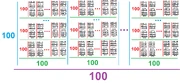11,052 Pages

The Hexfactor is equal to 100!6 using Extensible ! Notation. The term was coined by Yabuszko. This number is comparable to goggol and gulgol.Visual representation of hexfactor

## Etymology

The name of this number is based on Greek prefix "hexa-" and the factor.

## Approximations

Notation Approximation
Arrow Notation $$82\uparrow\uparrow\uparrow\uparrow\uparrow\uparrow101$$
Chained Arrow Notation $$82 \rightarrow 101 \rightarrow 6$$
BEAF $$\{82,101,6\}$$
Hyper-E notation

$$E160\#100\#\#5$$

Hyperfactorial Array Notation $$100!5$$
Strong Array Notation $$s(82,100,6)$$
Nested Factorial Notation $$100!$$
Fast-growing hierarchy $$f_7(100)$$
Hardy hierarchy $$H_{\omega^7}(100)$$
Slow-growing Hierarchy $$g_{\varphi(5,0)}(100)$$

## Sources

Community content is available under CC-BY-SA unless otherwise noted.